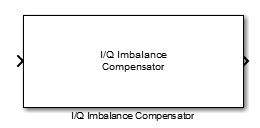Main Content

# I/Q Imbalance Compensator

Compensate for imbalance between in-phase and quadrature components

## Library

RF Impairments Correction

•## Description

The I/Q Imbalance Compensator mitigates the effects of an amplitude and phase imbalance between the in-phase and quadrature components of a modulated signal. The supported modulation schemes include OFDM, M-PSK, and M-QAM, where M > 2.

This block accepts up to three input ports, of which one is the input signal. When you set the Source of compensator coefficient parameter to ```Estimated from input signal```, two additional input ports are enabled. The first is enabled when you set the Source of adaptation step size parameter to `Input port` and the second is enabled when you check the Coefficient adaptation input port box. The two options are independent. Additionally, you can check the Estimated coefficient output port box to create an optional output port from which the estimated compensator coefficients are made available.

When you set the Source of compensator coefficient parameter to `Input port`, only one possible configuration is possible (input signal port, coefficient input port, and output signal port).

## Parameters

Source of compensator coefficient

Specify the source of the compensator coefficients as ```Estimated from input signal``` or `Input port`. If set to `Estimated from input signal`, the compensator calculates the coefficients from the input signal. If set to `Input port`, all other properties are disabled and you must provide the coefficients through the input port. The default value is `Estimated from input signal`.

Initial compensator coefficient

Specify the initial coefficient used by the internal algorithm to compensate for the I/Q imbalance. The default value is `0+0j`.

Source of adaptation step size

Specify the source of the adaptation step size as `Property` or ```Input port```. If set to `Property`, specify the step size in the Adaptation step size field. If set to `Input port`, you must specify the step size through an input port. The default value is `Property`.

Adaptation step size

Specify the step size of the adaptation algorithm as a real scalar. This parameter is available only when Source of adaptation step size is set to `Property`. The default value is `0.00001`.

Coefficient adaptation input port

Select this check box to create an input port that permits a signal to control the adaptation process. If the check box is selected and if the input signal is `true`, the estimated compensation coefficients are updated. If the adaptation port is not enabled or if the input signal is `false`, the compensation coefficients do not change. By default, the check box is not selected.

Estimated coefficient output port

Select this check box to provide the estimated compensation coefficients to an output port. By default, the check box is not selected.

## Algorithms

This block implements the algorithm, inputs, and outputs described on the `comm.IQImbalanceCompensator` reference page. The object properties correspond to the block parameters.

## Supported Data Types

PortSupported Data Types
Signal Input
• Double-precision floating point

• Single-precision floating point

Signal Output
• Double-precision floating point

• Single-precision floating point

Step Size
• Double-precision floating point

• Single-precision floating point

Adaptation
• Logical

Input Coefficients
• Double-precision floating point

• Single-precision floating point

Output Coefficients
• Double-precision floating point

• Single-precision floating point

 Anttila, L., M. Valkama and M. Renfors. "Blind Compensation of Frequency-Selective I/Q Imbalances in Quadrature Radio Receivers: Circularity-Based Approach." Proc. IEEE ICASSP. 2007, pp. III-245 -III-248.

 Kiayani, A., L. Anttila, Y. Zou, and M. Valkama, "Advanced Receiver Design for Mitigating Multiple RF Impairments in OFDM Systems: Algorithms and RF Measurements." Journal of Electrical and Computer Engineering. Vol. 2012.

## Support

#### Bridging Wireless Communications Design and Testing with MATLAB

Download white paper